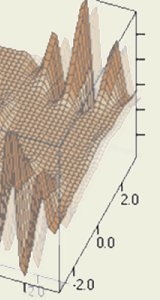#### Mathematical graph and charting software for geometry and statistics

The calculator can do statistics, best fits, function plotting, integration. It handles vectors, matrices, complex numbers, quaternions, coordinates, regular polygons and intersections.

For point, line, plane, sphere, circle Calc 3D calculates distances, intersections, and some additional information like volume and area.

Cartesian, spherical and cylindrical coordinates can be transformed into each other.

Carthesian plot, polar plot, parametric plot, best fit, fast fourier transformation, histogram, smooth,...

Several languages can be handeled.

• Look at the overview of features.
• Make a tour through Calc 3D.